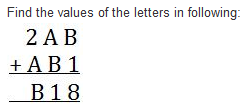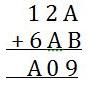Courses

Test: Playing With Numbers- 2

20 Questions MCQ Test Mathematics (Maths) Class 8 | Test: Playing With Numbers- 2

Description
Attempt Test: Playing With Numbers- 2 | 20 questions in 20 minutes | Mock test for Class 8 preparation | Free important questions MCQ to study Mathematics (Maths) Class 8 for Class 8 Exam | Download free PDF with solutions
QUESTION: 1

If the three digit number 24x is divisible by ‘9’, what is the value of ‘x’?

Solution:

For a number to be divisible by 9, the sum of its digits must be divisible by 9.

Here, 2 + 4 = 6. Next multiple of 9 is 9.

Therefore, we will add 9 -  6 = 3. So,  the value of x = 3.

QUESTION: 2

Solution:
QUESTION: 3

By which of the following number 9042 is not divisible? 2, 3, 6, and 9

Solution: Correct answer is'C' because the sum of 9042 is not a multiple of 9.
QUESTION: 4

Identify the missing digit in the number 234,4_6, if the number is divisible by 4.

Solution:
QUESTION: 5

Determine if 48 is divisible by 2, 3 and 5.

Solution: Yes, the B option is right because if you divide 48 by 2 and 3 it is easy to divide and reminder is 0 but if you divide 48 by 5 it is not divide. Thats why 48 is divisible by 2 and 3.
QUESTION: 6

By which of these numbers is the number 3116365 divisible?

Solution:

The last digit is 5, the number is divisible by 5.

Since

311636 − 10 = 311626 ÷ 7 = 44568,

the number is divisible by 7 also.

QUESTION: 7

Write in generalised form: 25

Solution:
QUESTION: 8

Which of the following statements is false?

Solution:

A number divisible by 3 and 4 is divisible by 12.

QUESTION: 9

If the division N ÷ 5 leaves a remainder of 0, what might be the one’s digit of N?

Solution:

Solution :- If a number is divisible by 5 then it's unit digit must be 0 or 5. so if we need the remainder of 0 when divided by 5 then the numbers unit digit must be 0 or 5.

QUESTION: 10

Write in the usual form: 10×5 + 6

Solution:

10 ×5 = 50
6 = 6
⇒ 50 + 6 = 56

QUESTION: 11Solution:

B+1=8
B=7, A+7=-1 .So A=4 and B=7

QUESTION: 12

The number 21436587 is divisible by _____.

Solution:
QUESTION: 13

Write in generalised form: 73

Solution:
QUESTION: 14

Write in the usual form: 100 × 7 + 10 × 1 + 8

Solution:
QUESTION: 15

Which of these numbers is divisible by 6?

Solution:

Divisible by 2 and 3 both.

QUESTION: 16

If the division N ÷ 2 leaves no remainder (i.e., zero remainder), what might be the one’s digit of N?

Solution:

Since every multiple of 2 leaves no remainder when divided by 2.Therefore N can be ,0,2,4,6 or 8

QUESTION: 17

Find the value of the letters in following:Solution:
QUESTION: 18

Write in generalised form: 85

Solution:
QUESTION: 19

Write in the usual form: 100 × a + 10 × c + b

Solution:

We can write acb=100*a+10*c+b which say that a is at hundred’s place, c is at ten’s place and b is at one’s place.

QUESTION: 20

If the division N ÷ 5 leaves a remainder of 4, what might be the one’s digit of N?

Solution:

Since every multiple of 5 ends with either 5 or 0. Therefore, the unit digit of N must be either 0+4=4 or 5+4=9. Hence the answer is either 4 or 9.Use Code STAYHOME200 and get INR 200 additional OFF Use Coupon Code

Track your progress, build streaks, highlight & save important lessons and more!

Similar ContentRelated tests